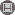Volume 6, Issue 1 (20 2010)                   irje 2010, 6(1): 46-50 | Back to browse issues page

BibTeX | RIS | EndNote | Medlars | ProCite | Reference Manager | RefWorks
Send citation to:Zare N, Sayadi M, Rezaeyan Fard E, Ghaem H. Application of Generalized Poisson Regression Model in Feminine Fertility Data Analysis in Rural Areas of Fars Province. irje. 2010; 6 (1) :46-50
URL: http://irje.tums.ac.ir/article-1-93-en.html
Abstract:   (12498 Views)
Background & objectives: statistical modeling explicates the observed changes in data by means of mathematics equations. In cases that dependent variable is count, Poisson model is applied. If Poisson model is not applicable in a specific situation, it is better to apply the generalized Poisson model. So, our emphasis in this study is to notice the data structure, introducing the generalized Poisson regression model and its application in estimates of effective factors coefficients on the number of children and comparing it with Poisson regression model results.
Methods: Besides introducing Poisson regression model, we introduced its application in fertility data analysis. A sample of 1019 women in rural areas of Fars was selected by cross sectional and stratified sampling methods. The number of children of family was determined as a count response variable for model validation.
Results: The sample mean and sample variance of the response variable Y, the number of children, are respectively 4.3 and 8.3 (over-dispersion). Log-likelihood was -1950.93 for Poisson regression and -1946.93 for generalized Poisson regression model.
Conclusions: The results revealed that this data have over-dispersion. According to selection criteria, the suitable model for this data analysis was generalized Poisson regression model. It can estimate effective factors coefficients on the number of children exactly.